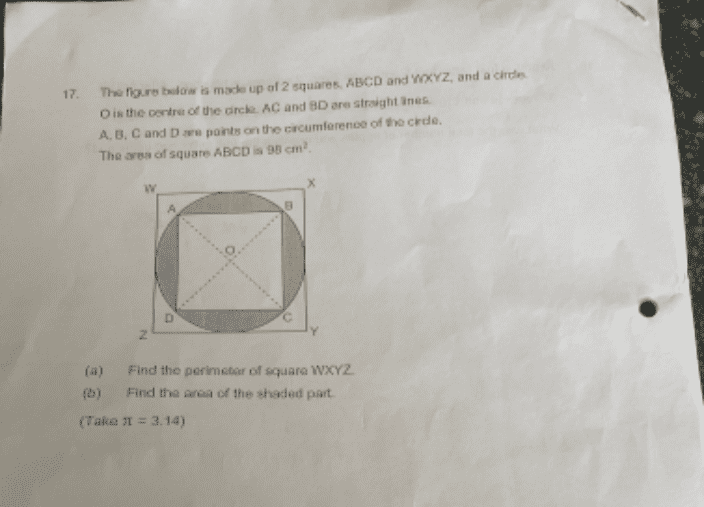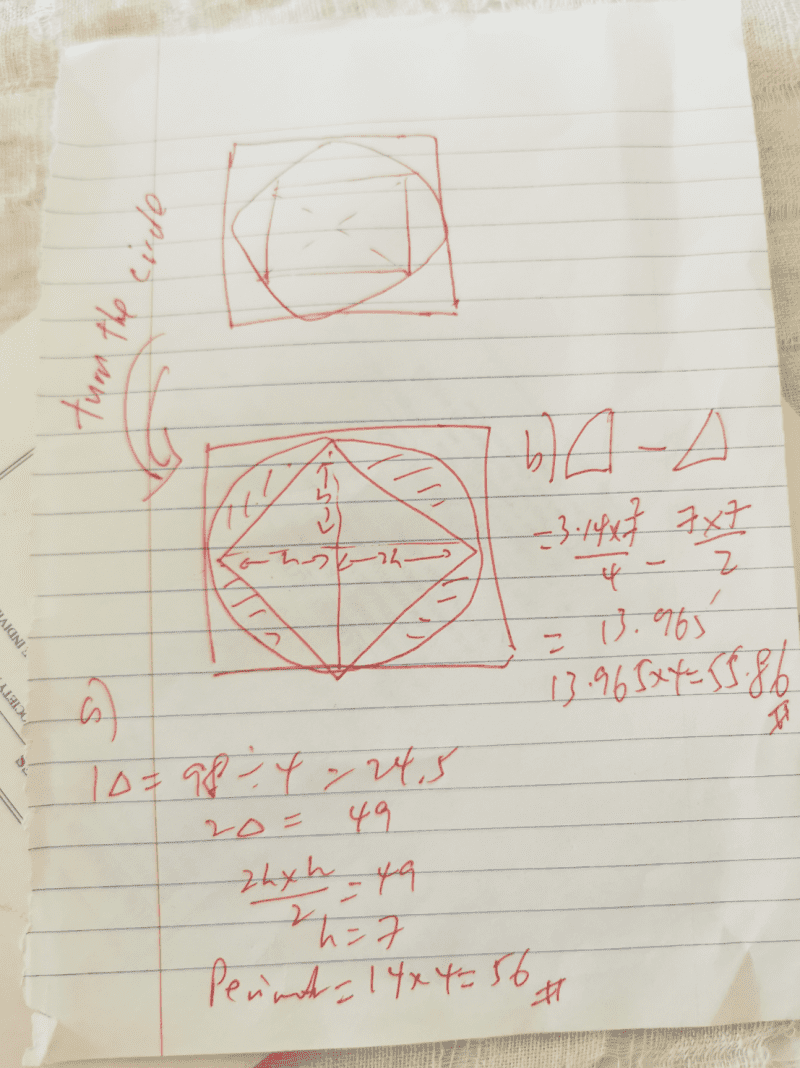# QuestionArea of ABCD = 98cm²
Now, AC = ✓(AB² + BC²) = ✓2AB² =  ✓(2×98) = 14cm

a) Since AC = WX,
Perimeter of WXYZ = 4×14 = 56 #

b) Area of circle = 3.14 x (14/2)² = 153.86cm²
Therefore area of shaded parts = 153.86 – 98 = 55.86cm² #

P6 no learn pythagoras theorem leh

btw ABCD area is 95 or 98